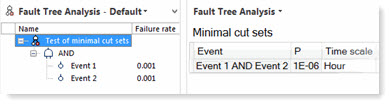While the Fault Tree Analysis Graph is used for viewing and editing fault trees, the Fault Tree Analysis Grid displays the so-called minimal cut sets of a tree, i.e., the smallest combination of events that lead to the top event, along with the corresponding probability of each event or combination of events. With the Fault Tree Analysis view open, switch to the grid by selecting the Grid tab at the bottom of the view.The grid includes columns that display the name of the set (Expression), the event probability rate (P) and the Time scale used.The event rate (P) and Time scale are attributes on the events called Failure rate and Failure rate time scale

The calculated probability (P) is the likelihood of a fault, normalized to the time scale used for the failure rates, as indicated by the Failure rate time scale attribute of the events.
In case different time scales are used for the events in a tree, joint probabilities cannot be calculated, and this is indicated in the grid by the use of a red font in the Time scale column:The probability of external events, if they are included in the fault tree, is included in the calculation of the probability of the top (fault) event.

The Grid tab works in the same way as the graph tab in that the view is generated for the item currently selected in the structure tree. You can sort by any column by clicking on the column header.

Note: The probability calculation is performed correctly even if the same event occurs multiple times in the tree as a common cause. For example, the joint probability of the two independent events "Event 1" and "Event 2" is calculated as the product of the separate probabilities:In case the same event ("Event 1" in the example) is used two times as input to the top AND condition, the calculated joint probability is equal to the single "Event 1" probability:# Modifying the Failure Rates and Failure Rate Time Scales

The event rate (P) and Time scale in the grid are default attributes on events. To modify either attribute for an event, select it and open the Overview or Attributes view. You can make your updates there.Other default attributes to meet your needs can be added to events as well, as shown in the above example.